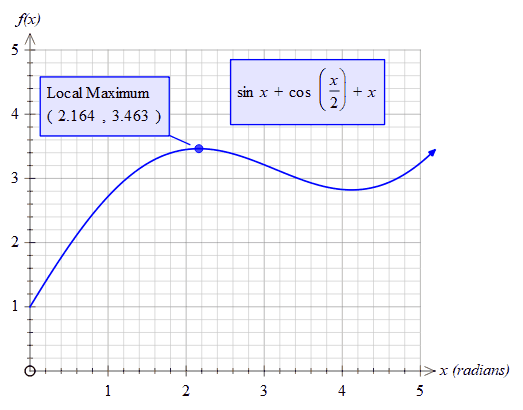﻿ fnMaxVal

# fnMaxVal

Top  Previous  Next
 fnMaxVal(function, low, high)   Finds the maximum y value  between low and high. For example:fnmaxval(sinx+cos(x/2)+x,1,3) = 3.463 fnmaxval(sinx+cos(x/2)+x,0,1) = 2.735   To find the x value of the local maximum, use the fnmax function